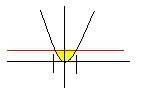# Reversing Double Integral

## Homework Statement

Evaluate an iterated integral by reversing the order of integration
$$\int^1_0\int^1_{y^2} ysin(x^2)dxdy$$

## The Attempt at a Solution

I've got that the limits for x is between y^2 and 1, while the limits for y is between 0 and 1. Then I graphed it:Looking at it from bottom to top, I see that it enters the region at y^2 and leaves at 1. While from left to right, the lowest limit x can be is -1, while the highest is 1. So now I have an integral of $$\int^1_{-1}\int^1_{\sqrt{x}}ysin(x^2)dydx$$.
Integrating the first part of the equation for y, I get:
$$\int^1_{-1} \frac{sin(x^2)}{2}-\frac{xsin(x^2)}{2}dx$$
And it's at this point that I get stuck. I know that I can break up the problem and integrate each part separately, which makes solving the second part easy, since I can just use substitution, but I'm just not sure how to integrate the $$\frac{sin(x^2)}{2}$$ part. I'm wondering whether this means that I got the limits wrong, or I'm just forgetting trig integrals.

What curves is the region in your question bounded by? I don't believe you included that and it makes it difficult to check what your limits of integration should be.

As I'm not sure what region you want the limits of integration for, It seems to me from your graph that you chose the correct ones. Have you tried Taylor expanding the first time to the desired degree of accuracy?

Last edited:
HallsofIvy
Homework Helper
Your picture is wrong. The area over which you are integrating is NOT the are you have shown in yellow. With x going from y2 to 1, you want to integrate over the region outside the parabola to the line x= 1 on the right.

Your picture is wrong. The area over which you are integrating is NOT the are you have shown in yellow. With x going from y2 to 1, you want to integrate over the region outside the parabola to the line x= 1 on the right.

Thanks, that solves the problem! Although, I can understand where I went wrong with taking the limits for x, but I still don't quite get why the region is the area outside the parabola, instead of inside.

HallsofIvy
Homework Helper
The second integral has lower limit x= y2 and upper limit x= 1. That order is verified by the fact that for y from 0 to 1 y2<= 1 so that is from x= y2 UP to x= 1.

The second integral has lower limit x= y2 and upper limit x= 1. That order is verified by the fact that for y from 0 to 1 y2<= 1 so that is from x= y2 UP to x= 1.

I don't know, I still don't get it. I mean yeah I understand the x-limits, it's just the y-limits I'm having problems with. Referring to the original integral, we have the limits of x being greater than y^2 and less than 1, so wouldn't that make the region above the parabola, since it has to be greater than the parabola?

HallsofIvy
NO!!! "x> y2" (x positive) means "$\sqrt{x}> y$" or $y< \sqrt{x}$.